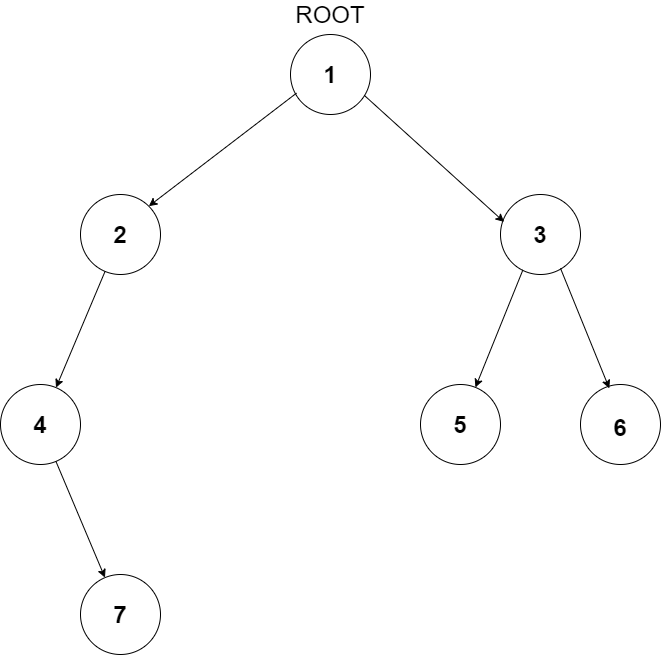# Longest Univalue Path | CodeStudio Solution

## Longest Univalue Path | CodeStudio Solution

Problem Statement
Suggest Edit

#### For example, consider the following binary tree:

``````            7
/ \
7   7
/ \   \
8  3    7
``````

#### For the above tree, the length of the longest path where each node in the path has the same value is 3 and path is 7 -> 7 -> 7 -> 7.

##### Input Format:
``````The first line of input contains an integer ‘T’ representing the number of test cases. Then the test cases follow.

The only line of each test case contains elements in the level order form. The line consists of values of nodes separated by a single space. In case a node is null, we take -1 on its place.

For example, the input for the tree depicted in the below image would be:
````````````1
2 3
4 -1 5 6
-1 7 -1 -1 -1 -1
-1 -1
``````

#### Explanation:

``````Level 1:
The root node of the tree is 1

Level 2:
Left child of 1 = 2
Right child of 1 = 3

Level 3:
Left child of 2 = 4
Right child of 2 = null (-1)
Left child of 3 = 5
Right child of 3 = 6

Level 4:
Left child of 4 = null (-1)
Right child of 4 = 7
Left child of 5 = null (-1)
Right child of 5 = null (-1)
Left child of 6 = null (-1)
Right child of 6 = null (-1)

Level 5:
Left child of 7 = null (-1)
Right child of 7 = null (-1)

The first not-null node(of the previous level) is treated as the parent of the first two nodes of the current level. The second not-null node (of the previous level) is treated as the parent node for the next two nodes of the current level and so on.
The input ends when all nodes at the last level are null(-1).
``````
##### Note:
``````The above format was just to provide clarity on how the input is formed for a given tree.
The sequence will be put together in a single line separated by a single space. Hence, for the above-depicted tree, the input will be given as:

1 2 3 4 -1 5 6 -1 7 -1 -1 -1 -1 -1 -1
``````
##### Output Format:
``````For each test case, a single integer denoting the length of the longest path where each node in the path has the same value is printed.

The output for each test case is to be printed on a separate line.
``````
##### Note:
``````You do not need to print anything; it has already been taken care of. Just implement the given function.
``````
##### Constraints:
``````1 <= T <= 100
1 <= N <= 3000
-10^9 <= data <= 10^9 and data != -1

Where ‘T’ is the number of test cases, ‘N’ is the total number of nodes in the binary tree, and “data” is the value of the binary tree node.

Time Limit: 1sec
``````
##### Sample Input 1:
``````2
7 7 7 1 1 -1 7 -1 -1 -1 -1 -1 -1
10 3 4 3 3 -1 5 -1 -1 -1 -1 -1 -1
``````
##### Sample Output 1:
``````3
2
``````
##### Explanation Of Sample Input 1:
``````            7
/ \
7   7
/ \   \
1  1    7

For the first test case, the length of the longest path where each node in the path has the same value is 3 and path is 7 -> 7 -> 7 -> 7.

10
/ \
3   4
/ \   \
3  3    5

For the second test case, the length of the longest path where each node in the path has the same value is 2 and path is 3 -> 3 -> 3.
``````
##### Sample Input 2:
``````2
1 4 5 4 4 -1 5 -1 -1 -1 -1 -1 -1
5 4 5 1 1 -1 5 -1 -1 -1 -1 -1 -1
``````
##### Sample Output 2:
``````2
2``````
``int ans=0;int helper(BinaryTreeNode<int>*root,int &curr){    if(!root)return 0;        int leftPath=helper(root->left,curr);    int rightPath=helper(root->right,curr);    int left=0,right=0;    if(root->left!=NULL  && root->left->data==root->data){        left+=leftPath+1;    }    if(root->right!=NULL && root->right->data==root->data){        right+=rightPath+1;    }    curr=max(curr,left+right);    return max(left,right);    }int longestUnivaluePath(BinaryTreeNode<int> *root) {    int curr=0;    helper(root,curr);    return curr;   }``